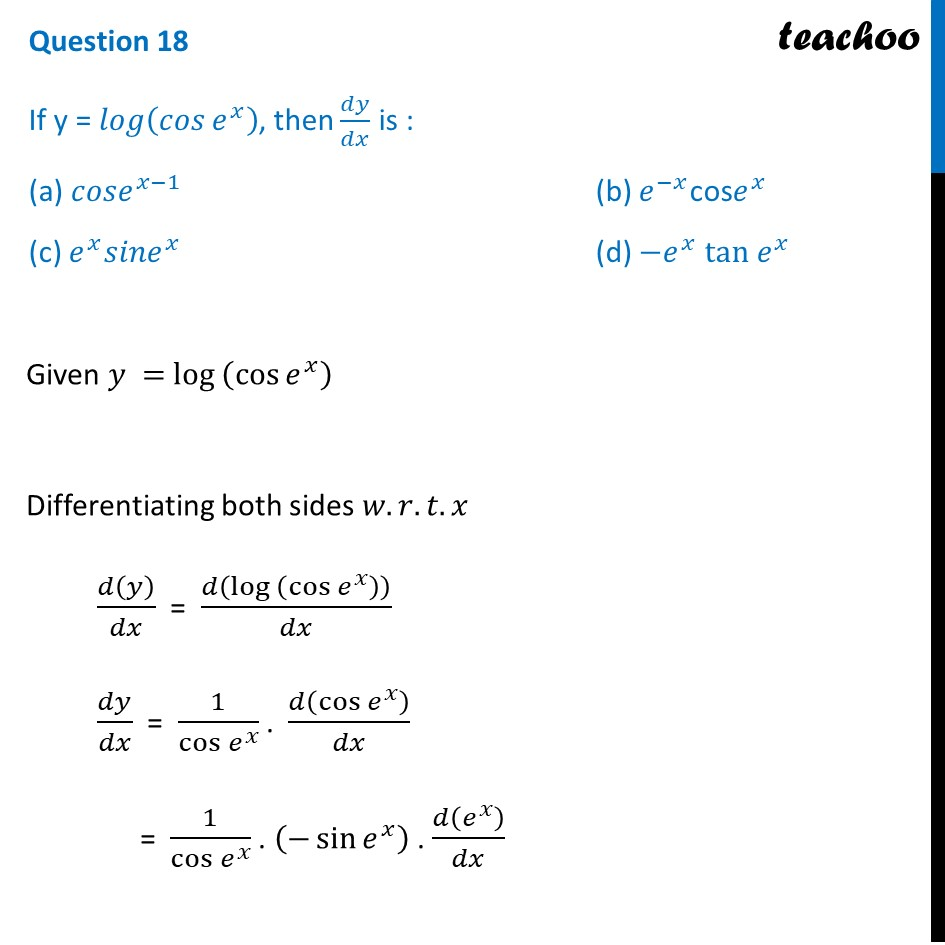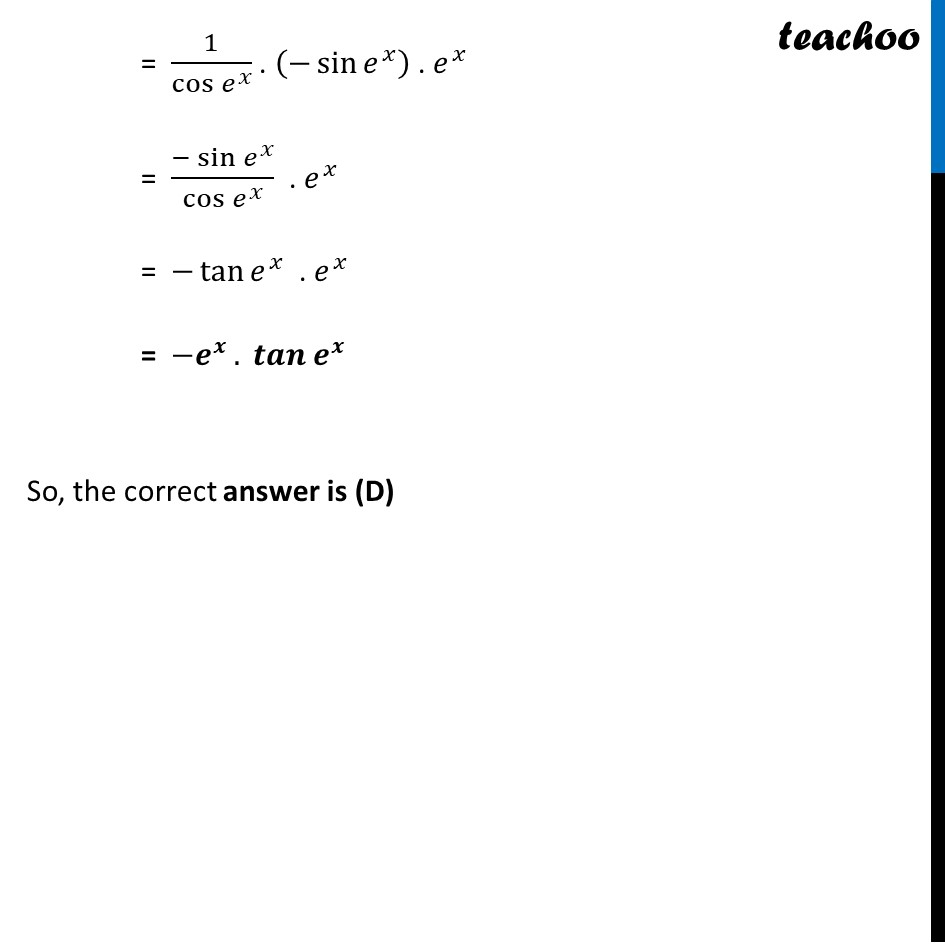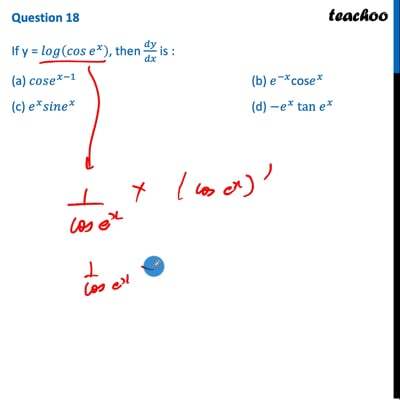CBSE Class 12 Sample Paper for 2022 Boards (MCQ Based - for Term 1)

Class 12
Solutions of Sample Papers and Past Year Papers - for Class 12 Boards

## (a) cose (x-1)   (b) e (-x) cos e x (c) e x sine x    (d) -e x tan e x

This question is inspired from Ex 5.4, 5 - Chapter 5 Class 12 - Continuity and DifferentiabilityThis video is only available for Teachoo black users

Introducing your new favourite teacher - Teachoo Black, at only ₹83 per month

### Transcript

Question 18 If y = 𝑙𝑜𝑔⁡(𝑐𝑜𝑠⁡〖𝑒^𝑥 〗 ), then 𝑑𝑦/𝑑𝑥 is : (a) 𝑐𝑜𝑠𝑒^(𝑥−1) (b) 𝑒^(−𝑥) "cos" 𝑒^𝑥 (c) 𝑒^𝑥 𝑠𝑖𝑛𝑒^𝑥 (d) 〖−𝑒〗^𝑥 " tan" 〖 𝑒〗^𝑥 Given 𝑦 = 〖log 〗⁡(cos⁡〖𝑒^𝑥 〗 ) Differentiating both sides 𝑤.𝑟.𝑡.𝑥 𝑑(𝑦)/𝑑𝑥 = 𝑑(〖log 〗⁡(cos⁡〖𝑒^𝑥 〗 ) )/𝑑𝑥 𝑑𝑦/𝑑𝑥 = 1/cos⁡〖𝑒^𝑥 〗 . 𝑑(cos⁡〖𝑒^𝑥 〗 )/𝑑𝑥 = 1/cos⁡〖𝑒^𝑥 〗 . (−sin⁡〖𝑒^𝑥 〗 ) . 𝑑(𝑒^𝑥 )/𝑑𝑥 = 1/cos⁡〖𝑒^𝑥 〗 . (−sin⁡〖𝑒^𝑥 〗 ) . 𝑒^𝑥 = (−sin⁡〖𝑒^𝑥 〗)/cos⁡〖𝑒^𝑥 〗 . 𝑒^𝑥 = −tan⁡〖𝑒^𝑥 〗 . 𝑒^𝑥 = −𝒆^𝒙 . 𝒕𝒂𝒏⁡〖𝒆^𝒙 〗 So, the correct answer is (D)